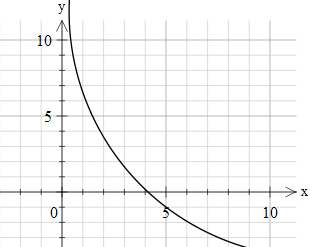# Logarithms, Trigonometry, Integrals, Part 2

Calculus Level 5$\displaystyle \int _{0}^{\pi/2} \ln(\sin x) \ln(\tan x) \space \mathrm{d}x$

Given that the above integral equals to $\frac {\pi^A}{B}$ for positive integers $A,B$, what is the value of $A+B$?

This is original. Check out my Set

×